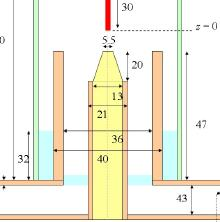# Technical Papers and Presentations

#### Study Of An Axial Injection Torch

K. Gadonna, O. Leroy, C. Boisse-Laporte, P. Leprince, and L.L. Alves
LPGP, CNRS/UPS, Orsay, France
IPFN/IST, Lisboa, Portugal
Published in 2010

The axial injection torch (AIT) produces high-density plasmas by coupling microwave power to a flowing gas. The plasma is imposed by defining its electron density and temperature whose maximum are given by optical emission spectroscopy diagnostics. Our study of an AIT with COMSOL Multiphysics is for the following modules:
- electromagnetic (3D), to calculate the distribution of the electromagnetic field in the presence of plasma, by solving an equation for the electric field. - hydrodynamic (2D), to calculate the distribution of mass density, flow and temperature of the neutral gas particles Tg, in the presence of a plasma heating source, by solving the corresponding mass, momentum, and thermal energy balance equations for helium. Simulations will provide input data to a plasma code (1D), which solves the transport equations for electrons and ions, the electron mean energy balance equation and Poisson\'s equation.